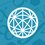# Finding the minimum sum

All numbers considered are positive real.

Given:

M spaces exist

In each space, N sets of the form (a,b,c) exist.

Now we have the following equations: $({a_{11}} + {b_{11}} + {c_{11}}) + ({a_{21}} + {b_{21}} + {c_{21}}) + ... + ({a_{N1}} + {b_{N1}} + {c_{N1}}) = {S_1}$ for space 1 … … $({a_{1M}} + {b_{1M}} + {c_{1M}}) + ({a_{2M}} + {b_{2M}} + {c_{2M}}) + ... + ({a_{NM}} + {b_{NM}} + {c_{NM}}) = {S_M}$ for space M

We know that the sum $S_Y$ is minimum. Then can we say that if we deduct a constant x from each middle element of each set of each space, then for the same combination of sets, we will get the minimum value? I.e. the new $S_{Y}$ will remain the minimum sum?

The new equations after deduction are: $({a_{11}} + {b_{11}} - x + {c_{11}}) + ({a_{21}} + {b_{21}} - x + {c_{21}}) + ... + ({a_{N1}} + {b_{N1}} - x + {c_{N1}}) = {S_1}$ … … $({a_{1M}} + {b_{1M}} - x + {c_{1M}}) + ({a_{2M}} + {b_{2M}} - x + {c_{2M}}) + ... + ({a_{NM}} + {b_{NM}} - x + {c_{NM}}) = {S_M}$Note by Santanu Banerjee
5 months ago

This discussion board is a place to discuss our Daily Challenges and the math and science related to those challenges. Explanations are more than just a solution — they should explain the steps and thinking strategies that you used to obtain the solution. Comments should further the discussion of math and science.

When posting on Brilliant:

• Use the emojis to react to an explanation, whether you're congratulating a job well done , or just really confused .
• Ask specific questions about the challenge or the steps in somebody's explanation. Well-posed questions can add a lot to the discussion, but posting "I don't understand!" doesn't help anyone.
• Try to contribute something new to the discussion, whether it is an extension, generalization or other idea related to the challenge.

MarkdownAppears as
*italics* or _italics_ italics
**bold** or __bold__ bold
- bulleted- list
• bulleted
• list
1. numbered2. list
1. numbered
2. list
Note: you must add a full line of space before and after lists for them to show up correctly
paragraph 1paragraph 2

paragraph 1

paragraph 2

[example link](https://brilliant.org)example link
> This is a quote
This is a quote
    # I indented these lines
# 4 spaces, and now they show
# up as a code block.

print "hello world"
# I indented these lines
# 4 spaces, and now they show
# up as a code block.

print "hello world"
MathAppears as
Remember to wrap math in $$ ... $$ or $ ... $ to ensure proper formatting.
2 \times 3 $2 \times 3$
2^{34} $2^{34}$
a_{i-1} $a_{i-1}$
\frac{2}{3} $\frac{2}{3}$
\sqrt{2} $\sqrt{2}$
\sum_{i=1}^3 $\sum_{i=1}^3$
\sin \theta $\sin \theta$
\boxed{123} $\boxed{123}$

Sort by:

I suppose the answer is obviously yes. Remove the $x$ from the brackets, and you would get $nx$. Since $S_Y\ge S_{\text{random}}$, $S_Y-nx\ge S_{\text{random}}-nx$ wouldn’t it? :)

- 5 months ago